## NCERT Solutions for Class 11 Maths Chapter 6 Linear Inequalities Exercise 6.2

Chapter 6 Linear Inequalities Exercise 6.2 NCERT Solutions for Class 11 Maths that will be useful in knowing the basic concepts of the chapter and easily solve difficult questions. If you ever have doubt regarding any question then you can take help of this page. Class 11 Maths NCERT Solutions will also help you in making your own answers.1. Represent the following inequality graphically in a two dimensional plane.
x + y < 5

We draw the graph of the equation
x + y = 5 ...(i)
Putting y = 0, x = 5, therefore the point on the x-axis is (5, 0). The point on the y-axis is (0, 5). AB is the graph of (i) (See Fig)Putting x = 0, y = 0 in the given inequality, we have 0 + 0 < 5 or 5 > 0 which is true. Hence, origin lies in the half plane region I.
Clearly, any point on the line does not satisfy the given inequality.
Hence, the shaded region I excluding the points on the line is the solution region of the inequality.

2. Represent the following inequality graphically in a two dimensional plane. 2x + y ≥ 6

We draw the graph of the equation
2x + y = 6 ...(i)
Putting x = 0, y = 6, therefore the point on y-axis is (0, 6) and the point on x-axis is (3, 0). AB is the graph of (i).
Putting x = 0, y = 0 in the given inequality, we have
2(0) + 0 ≥ 6 or 0 ≥  6, which is false.
Hence, origin does not lie in the half plane region I.
Clearly, any point on the line satisfy the given inequality.Hence, the shaded region II including the points on the line is the solution region of the inequality.

3. Solve the inequality 3x + 4y ≤ 12 graphically in two-dimensional plane.

We draw the graph of the equation 3x + 4y = 12. The line passes through the points (4, 0), (0, 3). This line is represented by AB.
Now consider the inequality 3x + 4y ≤ 12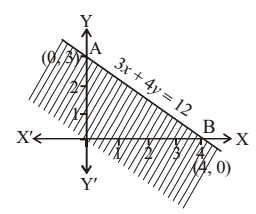Putting x = 0, y = 0
0 + 0 = 0 ≤ 12, which is true
∴ Origin lies in the region of 3x + 4y ≤ 12
The shaded region represents this inequality.

4. Find the solutions of following inequality graphically in a two dimensional plane.
y + 8 ≥ 2x

We draw the graph of the equation
y + 8 = 2x
or y – 2x = – 8
or 2x – y = 8 ...(i)
Putting x = 0, y = – 8, therefore the point on the y-axis is (0, – 8). The point on the x-axis is (4, 0). AB is the graph of (i).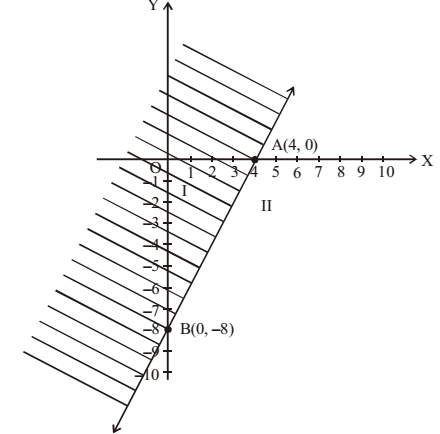Putting x = 0, y = 0 in the given inequality we have 0 + 8 ≥ 2 (0) or 8 ≥ 0, which is true. Hence origin lies in the half plane region I.
Clearly, any point on the line satisfy the given inequality.
Hence, the shaded region I including the points on the line is the solution region of the inequality.

5. Find the solutions of following inequality graphically in a two dimensional plane.
x – y ≤ 2

We draw the graph of the equation
x – y = 2 ...(i)Putting x = 0, y = – 2, therefore the point on the y-axis is (0, –2). The point on the x-axis is (–2, 0). AB is the graph of (i).
Putting x = 0, y = 0 in the given inequality, we have 0 – 0 ≤ 2 or 0 ≤ 2, which is true. Hence origin lies in the half plane region I.
Clearly, all the points on the line satisfy the given inequality.
Hence, the shaded region I including the points on the line is the solution region of the inequality.

6. Solve the following inequality graphically in two dimensional plane:
2x – 3y > 6

We draw the graph of 2x – 3y = 6
The line passes through (3, 0), (0, –2)
AB represents the equation 2x – 3y = 6
Now consider the inequality 2x – 3y > 6
Putting x = 0, y = 0
0 = 0 > 6 is not true.
∴ Origin does not lie in the region of
2x – 3y > 6
The graph of 2x – 3y > 6 is shown as shaded area.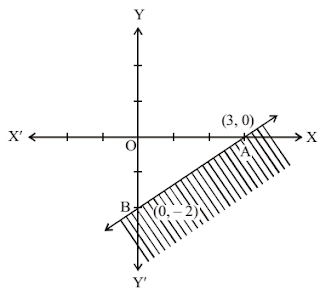7. Solve the inequality –3x + 2y ≥ –6 graphically in two-dimensional plane

Let us draw the line –3x + 2y = –6
The line passes through the points (2, 0), (0, –3)
The line AB represents the equation
– 3x + 2y = – 6Now, consider the inequality -3x + 2y ≥ - 6
Putting x = 0, y = 0
-0 + 0 ≥ -6, which is true
Origin lies in the area denoted by the inequality -3x + 2y ≥ -6. Graph of this inequality is shown in the figure by shaded area.

8. Solve the given inequality 3y – 5x < 30 graphically in two -dimensional plane.

Given inequality is 3y – 5x < 30 … (i)
Let us draw the graph of the line
3y – 5x = 30 … (ii)
Putting y = 0, x = –6.
Hence, the point on the X-axis is (–6, 0)
Again, putting x = 0, y = 10
Hence, the point on axis of y is (0, 10)
AB is the graph of equation (ii) (see figure)
Putting x = 0 and y = 0 in (i)
we have 0 – 0 < 30 or 30 > 0 which is true Therefore, origin lies in half plane region I.
Shaded portion (excluding the line AB) is the solution region.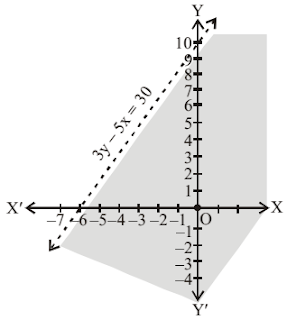9. Solve the following inequality graphically in two dimensional plane.
y < – 2

Given inequality is
y < – 2 ...(1)
Let us draw the graph of the line y = – 2 (see figure).
AB is the required line.
Putting y = 0 in (1), we have 0 < –2, which is not true.
The solution region is the shaded region below the line.
Hence, every point below the line (excluding the line) is the solution area.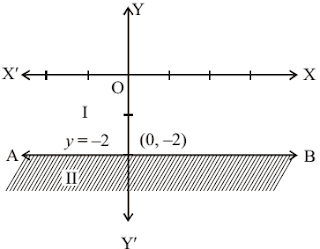10. Solve the following inequality graphically in two dimensional plane:
x > – 3